×#### Thank you for registering.

One of our academic counsellors will contact you within 1 working day.

Click to Chat

1800-1023-196

+91-120-4616500

CART 0

• 0

MY CART (5)

Use Coupon: CART20 and get 20% off on all online Study Material

ITEM
DETAILS
MRP
DISCOUNT
FINAL PRICE
Total Price: Rs.

There are no items in this cart.
Continue Shopping• Complete JEE Main/Advanced Course and Test Series
• OFFERED PRICE: Rs. 15,900
• View Details

```Chapter 20: Linear Equation in One Variable Exercise – 20.3

Question: 1

Find the area of the pentagon shown in figure below, if AD = 10 cm, AG = 8 cm, AH= 6 cm, AF= 5 cm, BF = 5 cm, CG = 7 cm and EH = 3 cm.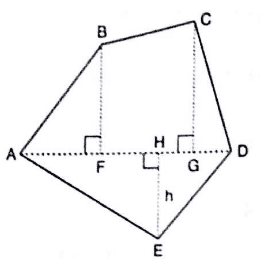Solution:

Given:

AD = 10 cm, AG = 8 cm, AH = 6 cm, AF = 5 cm

BF = 5 cm, CG = 7 cm, EH = 3 cm

Therefore, FG = AG – AF = 8 – 5 = 3 cm

And, GD = AD – AG = 10 – 8 = 2 cm

From given figure:Question: 2

Find the area enclosed by each of the following figures (Fig. 20.49 (i)-(iii)J as the sum of the areas of a rectangle and a trapezium: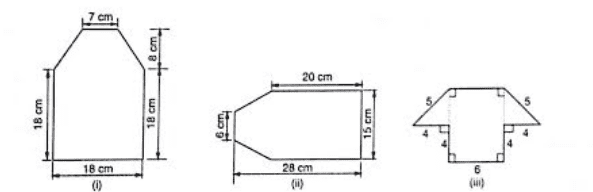Solution:

(i) From the figure:

Area of the complete figure = (Area of square ABCF) + (Area of trapezium CDEF)Question: 3

There is a pentagonally shaped park as shown in Fig. 20.50. Jyoti and Kavita divided it in two different ways.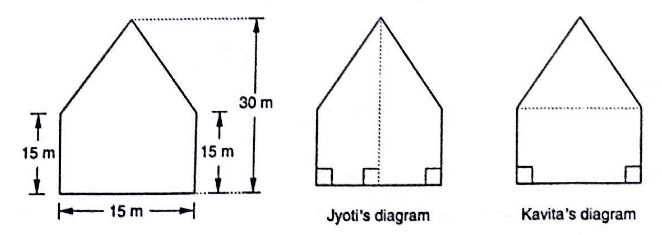Find the area of this park using both ways. Can you suggest some another way of finding its area?

Solution:

A pentagonal park is given below: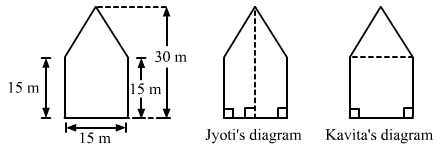Jyoti and Kavita divided it in two different ways.

(i) Jyoti divided is into two trapeziums. It is clear that the park is divided in two equal trapeziums whose parallel sides are 30 m and 15 m.Question: 4

Find the area of the following polygon, if AL =10 cm, AM = 20 cm, AN = 50 cm, AO = 60 cm and AD = 90 cm.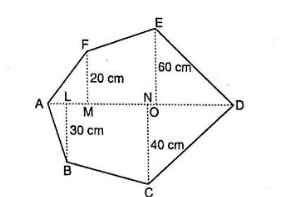Solution:

Given: AL = 10 cm, AM=20 cm, AN=50 cm

AO = 60 cm, AD = 90 cm

Hence, we have the following: MO = AO – AM = 60 – 20 = 40 cm

OD = AD – A0 = 90 – 60 = 30 cm

ND = AD – AN = 90 – 50 = 40 cm

LN = AN – AL = 50 – 10 = 40 cm

From given figure:

Area of Polygon = (Area of triangle AMF) + (Area of trapezium MOEF) + (Area of triangle DNC) + (Area of trapezium NLBC) + (Area of triangle ALB)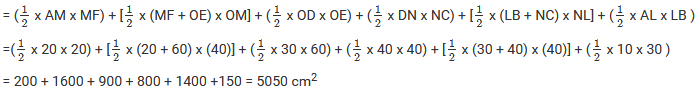Question: 5

Find the area of the following regular hexagon.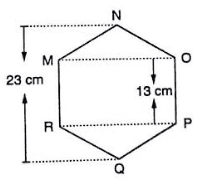Solution:

The given figure is :

Join QN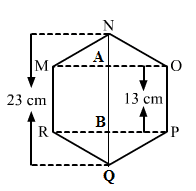It is given that the hexagon is regular. So, all its sides must be equal to 13 cm.

Also, AN = BQ

QB + BA + AN = QN

AN + 13 + AN = 23

2AN = 23 – 13 = 10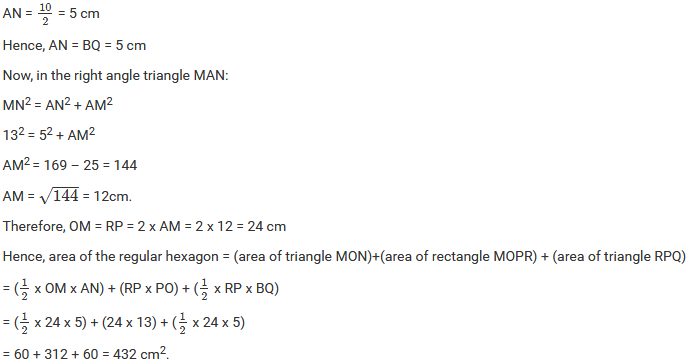```### Course Features

• 728 Video Lectures
• Revision Notes
• Previous Year Papers
• Mind Map
• Study Planner
• NCERT Solutions
• Discussion Forum
• Test paper with Video Solution